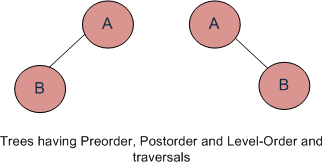# If you are given two traversal sequences, can you construct the binary tree?

• Difficulty Level : Easy
• Last Updated : 15 Jun, 2022

It depends on what traversals are given. If one of the traversal methods is Inorder then the tree can be constructed, otherwise not.Therefore, following combination can uniquely identify a tree.

• Inorder and Preorder.
• Inorder and Postorder.
• Inorder and Level-order.

And following do not.

• Postorder and Preorder.
• Preorder and Level-order.
• Postorder and Level-order.

For example, Preorder, Level-order and Postorder traversals are same for the trees given in above diagram.

• Preorder Traversal = AB
• Postorder Traversal = BA
• Level-Order Traversal = AB

So, even if three of them (Pre, Post and Level) are given, the tree can not be constructed.

My Personal Notes arrow_drop_up